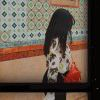{meta: 原调: 1=#D} {meta: 选调: 1=#C} {meta: Capo=2} {comment: 前奏/间奏} {comment: 主歌 A} 别[C]再 奋[G]力 逞[F]强 狂[C]欢 掩[G]饰 倔[F]强 面[C]对 空[G]洞 四[Am]面 堂[F]皇 有没[C]有 一[G]些 赔[F]偿 {comment: 主歌 B} 谁[C]把 星[G]星 点[F]亮 月[C]光 明[G]珠 万[F]丈 看[C]清 黑[G]夜 灯[Am]熄灭也 无[F]妨 因为 [C]风再凉伞 [G]还是在 身[Am]旁 就算 [C]风衣旧了 [G]仍把寒 阻[Am]挡 {comment: 副歌} {start_of_chorus} 璀[C]璨的年华在 [G]飘逸 我[Am]们忘了沾沾 [F]自喜 [C]最后 在[G]麦田里 相[Am]聚 （[F]会集） [C]别忘了 落[G]叶也归 [Am]地 [F]oh~ [C]我们 在[G]麦田里 相[Am]聚 [F]等你 璀[C]璨的年华在 [G]飘逸 我[Am]们忘了沾沾 [F]自喜 [C]最后 在[G]麦田里 相[Am]聚 （[F]会集） [C]别忘了 落[G]叶也归 [Am]地 [F]oh~ [C]我们 在[G]麦田里 会[Am]集 [F]光明 [C]是灰是白 [G]谁还在衡[Am]量[F] 记得[C]风衣带着 [G]记得把头[Am]昂[F] 璀[C]璨的年华在 [G]飘逸 我[Am]们忘了沾沾 [F]自喜 [C]最后 在[G]麦田里 相[Am]聚 [F]光明 [C]别忘了 [G]落叶也归[Am]地 [F]oh~ [C]我们 在[G]麦田里 云[Am]集 [F]极美 [C]欢庆 {end_of_chorus} {title:极美} {artist:孙燕姿} {author:poppy}DM